# The words of one often hide the words of others

Due to the constant growth of the volume of information, the need for data compression technologies is increasing. Compression multimedia and text data needed to save memory, pack additional data, and reduce communication time. One such method is arithmetic coding.

Arithmetic coding (AK) is a statistical data compression method that works on the principle of encoding one character at a time. The length of the encoded output code of each symbol may vary depending on the probability of the frequency of occurrence of the symbol. Fewer bits are used to encode characters that occur with high probability, and more bits that occur with low probability.

The idea of ​​the AK algorithm:

In arithmetic coding, a word is represented as an interval of real numbers from 0 to 1. As the length of a word increases, the interval for its representation decreases and the number of bits for its definition increases. More likely characters decrease the spacing by a smaller amount than less likely characters, and therefore add fewer bits to a word.

Description of the algorithm:

Example:

Let the alphabet be given A = {a, b}, m = 3. I will construct segments for all words of length mfrom the characters of the given alphabet.

Solution:

I will consider a set of all possible words of a given length m. Moreover, I will arrange the words in lexicographic order

where Ais an alphabet, with the number of characters equal to n.

Associate each word with a segment, the length of which coincides with its probability. On each segment, some arbitrary point is selected. Then, the code of the word will be a binary record of the coordinate of a point on the segment. The points must be chosen different so as not to violate the code separability condition. To minimize the code length, it is necessary to choose the point with the shortest binary notation. We will call this algorithm naive.

I will designate: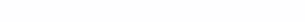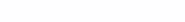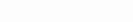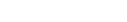Now that the segments of all words of length 3 are known, I will find the code of the word aab. But first, let me introduce the following notation:

B(α)-coordinate of the beginning of the word α

E(α)-coordinate of the end of the word α

B(aab) = 0.216; E(aab) = 0.36.

Convert numbers from decimal to binary:

Word code aab I choose a common prefix of the coordinates of the beginning and end in binary notation, namely 01since it will be the minimum length.

There are many implementations of the arithmetic coding algorithm on the Internet, and they differ slightly from each other. Below is a link to the repository with the implementation of the user Izakharov https://gist.github/com/Izakharov/31278ecb5159e7180f009c557e7a94a4.

I will be engaged in improvement, optimization of the “naive” arithmetic coding algorithm.

Naive Algorithm Optimization

Let me introduce additional definitions to the naive algorithm:

I will consider the interval for all words except the last one. For the last word, the spacing will be . This addition introduces more certainty into the algorithm, since now, for sure, it will be possible to avoid the case when the chosen points for different words coincide, which entails a violation of the code separability condition.

Code calculation algorithm:

Let the coordinates of the beginning and end of the word be known.

B(α)= 0,r_1 r_2…r_k 0…
E(α)= 0,r_1 r_2…r_k 1…

Then the code of the word α will be the common prefix of the coordinates of the beginning and end in binary notation, that is, 〖 r〗_1 r_2…r_k.

In the case when there is no common prefix, and this is possible only if B(α)<0.5 and E(α) ≥0.5, an empty string is chosen as the word code. In arithmetic decoding, it is necessary to use data on the length of words and the text as a whole, so that the empty string as a code does not cause any contradictions.

It is easy to derive formulas for finding the coordinates of the beginning and end of words.

α is some word, a_j is a symbol from the alphabet A.

Representing numbers as fractions with a denominator

In the program I will store only integers: numerators of fractions and exponents of the denominator (k and r). This condition is necessary in order to calculate the code, at least with a small error. If the arithmetic coding algorithm is implemented “on the forehead”, then the codes of long words will be calculated incorrectly as a result of the accumulation of errors.

Scale transformations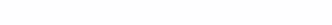1. I will assume that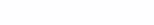Then, you can multiply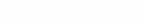by 2 and fix the obtained values. If, after further calculations, the answer is divided by 2, then it will not change. This is explained by the fact that under this condition, the first character of the code is guaranteed to be 0, because if you multiply two decimal numbers that are less than 0.5 by two, then the integer part will not appear, which means that the common prefix starts from zero.

2. GuessThen you can assign 1 to the code, subtract 1/2, multiply by 2 and continue the algorithm. Since the numbers are greater than 0.5, when multiplied by 2, they will become greater than one, therefore, a common prefix equal to one will appear.

Thus, it is possible not to convert numbers into binary notation, and even more so not to look for a common prefix, spending computational and time resources on this. Below is the code of the program that performs the arithmetic encoding of the input word.

``````A = 'abcd'
P = [2, 5, 5, 4]
r = 4

def coding(text):
beg, end = 0, 1
prob = 1
k = k1 = 0
code=""

for symbol in text:
pos = A.find(symbol)
end = beg*(2**r) + prob*sum(P[0:pos+1])
beg = beg*(2**r) + prob*sum(P[0:pos])
prob = prob*P[pos]
k += r
k1 += r

while True:
if end < 2**(k-1):
k -= 1
code += '0'
elif beg >= 2**(k-1):
beg -= 2**(k-1)
end -= 2**(k-1)
k -= 1
code += '1'
elif beg % 2 == 0 and end % 2 == 0:
beg /= 2
end /= 2
prob /= 2
k -= 1
elif k > 30:
beg //= 2
end //= 2
prob = end - beg
k -= 1
else:
break
return code``````

Program listing:

These two conditions are nothing but scaling transformations. Since only integers, numerator and denominator are stored in the program, the comparison is made not with 1/2, but with 2^(k-1)

``````if end < 2**(k-1):
k -= 1
code += '0'
elif beg >= 2**(k-1):
beg -= 2**(k-1)
end -= 2**(k-1)
k -= 1
code += '1'``````

This piece of code is used to simplify a fraction whose denominator is a number greater than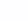``````elif k > 30:
beg //= 2
end //= 2
prob = end - beg
k -= 1``````

The purpose of the next condition is obvious. Here, if the numerator of a fraction is a multiple of two, then it is reduced by two, and the degree of the denominator is reduced by one. Such abbreviations make calculations easier and increase the speed of the program.

``````elif beg % 2 == 0 and end % 2 == 0:
beg /= 2
end /= 2
prob /= 2
k -= 1``````

Practical use

In this section of code, an input test message is compiled, which will then be encoded.

``````s=""
for i in range(1_000_000):
ln = random.randint(1, 7)
for j in range(ln):
s += random.choice('abcd')
s += ' '``````

Below, the input message is written to a file and then encoded using the arithmetic coding method.

``````with open('s.txt', 'w') as f:
f.write(s)

code = coding(s)``````

Having received a message code consisting of zeros and ones, you need to understand how much space it will take in the computer’s memory. To do this, it is translated into a sequence of bits and, for further comparison of the occupied memory, is written to a file.

``````pack = b''
for i in range(0, len(code), 8):
pack += struct.pack('B', int(code[i:i+8], 2))

with open('pack_code.txt', 'wb') as f:
f.write(pack)``````

To check that everything is done correctly, I will try to extract the byte stream from the file and convert it to source code.

``````with open('pack_code.txt', 'rb') as f:

code_unpack = ''
for i in unpack:
code_unpack += '{0:08b}'.format(i)

print(f'Is the original code equal to the code converted from bytes: {code_unpack == code}')``````

The result of the program